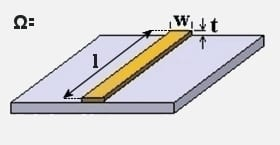Traces

## Trace Resistance CalculatorTrace Width
w
Trace Length
l
Trace Thickness
t
Temperature
Θ

### Output ⊕

Resistance:
0.364 Ω
Thermal resistance:
5.25 x 104 °C/Watt

View Notes

#### Calculation Notes

The resistance of a trace can be used to determine the power dissipation, and also to ascertain the affect on components such as precision resistors, where accurate resistance values are important.

Factors that influence the resistance calculation include:-

• Trace width (w)
• Trace length (l)
• Trace thickness (t)
• Temperature (Θ)

\rho = 1.724 \times 10^{-6}\,\Omega\,cm \; (copper resistivity)

\alpha = 3.93 \times 10^{-3}\;per\;^\circ C (temperature coefficient)

R = \rho \times \frac{l}{t \times w} \times \Big\{1+\big[\alpha\times \left(\Theta -20 \right) \big] \Big\}

References:
• Electrical Circuit Theory and Technology (ISBN 978-1-85617-770-2)
• Signal Integrity Issues and Printed Circuit Board Design (ISBN 0-13-335947-6)

Disclaimer: The information and this tool are provided with no liability of any kind whatsoever, use at your own risk.